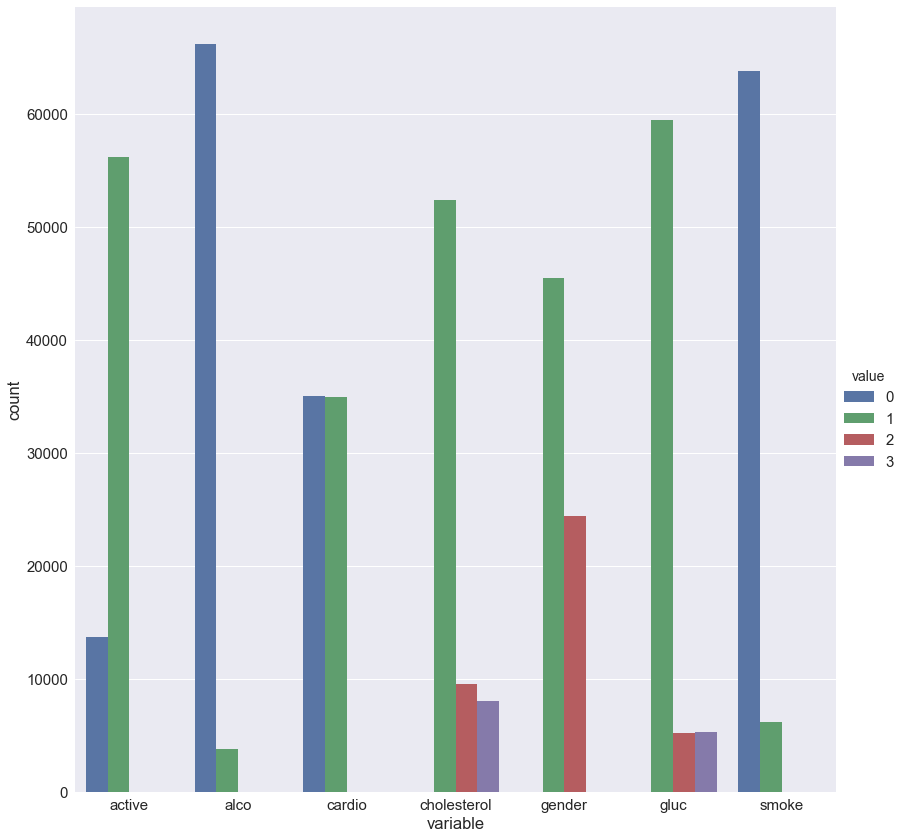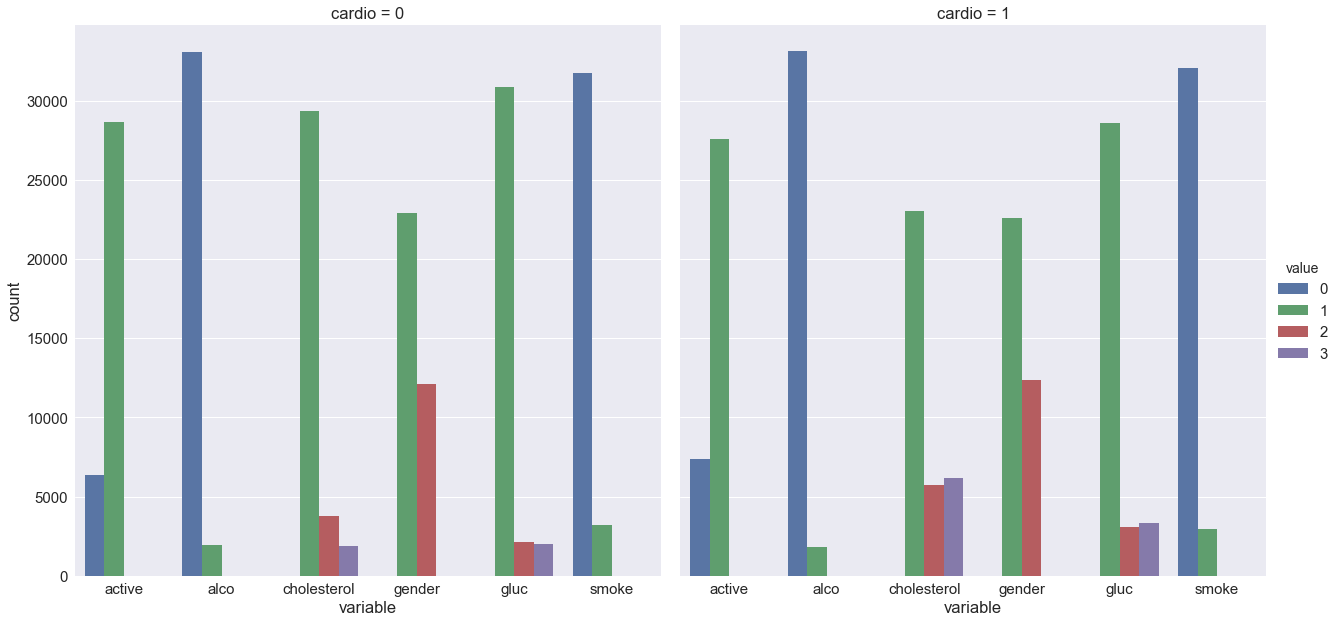## Open Machine Learning Course¶

Authors: Ilya Baryshnikov (ivi.ru), Maxim Uvarov (Teradata), and Yury Kashnitsky (Mail.Ru Group)
Translated and edited by Inga Kaydanova, Egor Polusmak, Anastasia Manokhina, and Yuanyuan Pao

All content is distributed under the Creative Commons CC BY-NC-SA 4.0 license.

# Assignment #2 (demo)

## Analyzing cardiovascular disease data

In this assignment, you will answer questions about a dataset on cardiovascular disease. You do not need to download the data: it is already in the repository. There are some Tasks that will require you to write code. Complete them and then answer the questions in the form.

#### Problem¶

Predict the presence or absence of cardiovascular disease (CVD) using the patient examination results.

#### Data description¶

There are 3 types of input features:

• Objective: factual information;
• Examination: results of medical examination;
• Subjective: information given by the patient.
Feature Variable Type Variable Value Type
Age Objective Feature age int (days)
Height Objective Feature height int (cm)
Weight Objective Feature weight float (kg)
Gender Objective Feature gender categorical code
Systolic blood pressure Examination Feature ap_hi int
Diastolic blood pressure Examination Feature ap_lo int
Cholesterol Examination Feature cholesterol 1: normal, 2: above normal, 3: well above normal
Glucose Examination Feature gluc 1: normal, 2: above normal, 3: well above normal
Smoking Subjective Feature smoke binary
Alcohol intake Subjective Feature alco binary
Physical activity Subjective Feature active binary
Presence or absence of cardiovascular disease Target Variable cardio binary

All of the dataset values were collected at the moment of medical examination.

Let's get to know our data by performing a preliminary data analysis.

# Part 1. Preliminary data analysis¶

First, we will initialize the environment:

In :
# Import all required modules
import pandas as pd
import numpy as np

# Disable warnings
import warnings
warnings.filterwarnings("ignore")

# Import plotting modules
import seaborn as sns
sns.set()
import matplotlib
import matplotlib.pyplot as plt
import matplotlib.ticker
%matplotlib inline


You will use the seaborn library for visual analysis, so let's set that up too:

In :
# Tune the visual settings for figures in seaborn
sns.set_context(
"notebook",
font_scale=1.5,
rc={
"figure.figsize": (11, 8),
"axes.titlesize": 18
}
)

from matplotlib import rcParams
rcParams['figure.figsize'] = 11, 8


To make it simple, we will work only with the training part of the dataset:

In :
df = pd.read_csv('../../data/mlbootcamp5_train.csv', sep=';')
print('Dataset size: ', df.shape)

Dataset size:  (70000, 13)

Out:
id age gender height weight ap_hi ap_lo cholesterol gluc smoke alco active cardio
0 0 18393 2 168 62.0 110 80 1 1 0 0 1 0
1 1 20228 1 156 85.0 140 90 3 1 0 0 1 1
2 2 18857 1 165 64.0 130 70 3 1 0 0 0 1
3 3 17623 2 169 82.0 150 100 1 1 0 0 1 1
4 4 17474 1 156 56.0 100 60 1 1 0 0 0 0

It would be instructive to peek into the values of our variables.

Let's convert the data into long format and depict the value counts of the categorical features using factorplot().

In :
df_uniques = pd.melt(frame=df, value_vars=['gender','cholesterol',
'gluc', 'smoke', 'alco',
'active', 'cardio'])
df_uniques = pd.DataFrame(df_uniques.groupby(['variable',
'value'])['value'].count()) \
.sort_index(level=[0, 1]) \
.rename(columns={'value': 'count'}) \
.reset_index()

sns.factorplot(x='variable', y='count', hue='value',
data=df_uniques, kind='bar', size=12);We can see that the target classes are balanced. That's great!

Let's split the dataset by target values. Can you already spot the most significant feature by just looking at the plot?

In :
df_uniques = pd.melt(frame=df, value_vars=['gender','cholesterol',
'gluc', 'smoke', 'alco',
'active'],
id_vars=['cardio'])
df_uniques = pd.DataFrame(df_uniques.groupby(['variable', 'value',
'cardio'])['value'].count()) \
.sort_index(level=[0, 1]) \
.rename(columns={'value': 'count'}) \
.reset_index()

sns.factorplot(x='variable', y='count', hue='value',
col='cardio', data=df_uniques, kind='bar', size=9);You can see that the distribution of cholesterol and glucose levels great differs by the value of the target variable. Is this a coincidence?

Now, let's calculate some statistics for the feature unique values:

In :
for c in df.columns:
n = df[c].nunique()
print(c)
if n <= 3:
print(n, sorted(df[c].value_counts().to_dict().items()))
else:
print(n)
print(10 * '-')

id
70000
----------
age
8076
----------
gender
2 [(1, 45530), (2, 24470)]
----------
height
109
----------
weight
287
----------
ap_hi
153
----------
ap_lo
157
----------
cholesterol
3 [(1, 52385), (2, 9549), (3, 8066)]
----------
gluc
3 [(1, 59479), (2, 5190), (3, 5331)]
----------
smoke
2 [(0, 63831), (1, 6169)]
----------
alco
2 [(0, 66236), (1, 3764)]
----------
active
2 [(0, 13739), (1, 56261)]
----------
cardio
2 [(0, 35021), (1, 34979)]
----------


In the end, we have:

• 5 numerical features (excluding id);
• 7 categorical features;
• 70000 records in total.

## 1.1. Basic observations¶

Question 1.1. (1 point). How many men and women are present in this dataset? Values of the gender feature were not given (whether "1" stands for women or for men) – figure this out by looking analyzing height, making the assumption that men are taller on average.

1. 45530 women and 24470 men
2. 45530 men and 24470 women
3. 45470 women and 24530 men
4. 45470 men and 24530 women

Question 1.2. (1 point). Which gender more often reports consuming alcohol - men or women?

1. women
2. men

Question 1.3. (1 point). What is the difference between the percentages of smokers among men and women (rounded)?

1. 4
2. 16
3. 20
4. 24

Question 1.4. (1 point). What is the difference between median values of age for smokers and non-smokers (in months, rounded)? You'll need to figure out the units of feature age in this dataset.

1. 5
2. 10
3. 15
4. 20

## 1.2. Risk maps¶

On the website for the European Society of Cardiology, a SCORE scale is provided. It is used for calculating the risk of death from a cardiovascular decease in the next 10 years. Here it is:Let's take a look at the upper-right rectangle, which shows a subset of smoking men aged from 60 to 65. (It's not obvious, but the values in the figure represent the upper bound).

We see the value 9 in the lower-left corner of the rectangle and 47 in the upper-right. This means that, for people in this gender-age group whose systolic pressure is less than 120, the risk of a CVD is estimated to be 5 times lower than for those with the pressure in the interval [160,180).

Let's calculate that same ratio using our data.

Clarifications:

• Calculate age_years feature – round age to the nearest number of years. For this task, select only the people of age 60 to 64, inclusive.
• Cholesterol level categories differ between the figure and our dataset. The conversion for the cholesterol feature is as follows: 4 mmol/l $\rightarrow$ 1, 5-7 mmol/l $\rightarrow$ 2, 8 mmol/l $\rightarrow$ 3.
In :
# You code here


Question 1.5. (2 points). Calculate the fraction of the people with CVD for the two segments described above. What is the ratio of these two fractions?

1. 1
2. 2
3. 3
4. 4

## 1.3. Analyzing BMI¶

Create a new feature – BMI (Body Mass Index). To do this, divide weight in kilogramms by the square of the height in meters. Normal BMI values are said to be from 18.5 to 25.

In :
# You code here


Question 1.6. (2 points). Choose the correct statements:

1. Median BMI in the sample is within the range of normal BMI values.
2. The BMI for women is on average higher than for men.
3. Healthy people have, on average, a higher BMI than the people with CVD.
4. For healthy, non-drinking men, BMI is closer to the norm than for healthy, non-drinking women

## 1.4. Cleaning data¶

We can see that the data is not perfect. It contains "dirt" and inaccuracies. We'll see this better as we visualize the data.

Filter out the following patient segments (we consider these as erroneous data)

• diastolic pressure is higher than systolic
• height is strictly less than 2.5 percentile (Use pd.Series.quantile to compute this value. If you are not familiar with the function, please read the docs.)
• height is strictly more than 97.5 percentile
• weight is strictly less than 2.5 percentile
• weight is strictly more than 97.5 percentile

This is not everything that we can do to clean this data, but this is sufficient for now.

In :
# You code here


Question 1.7. (2 points). What percent of the original data (rounded) did we throw away?

1. 8
2. 9
3. 10
4. 11

# Part 2. Visual data analysis¶

## 2.1. Correlation matrix visualization¶

To understand the features better, you can create a matrix of the correlation coefficients between the features. Use the initial dataset (non-filtered).

Plot a correlation matrix using heatmap(). You can create the matrix using the standard pandas tools with the default parameters.

In :
# You code here


Question 2.1. (1 point). Which pair of features has the strongest Pearson's correlation with the gender feature?

1. Cardio, Cholesterol
2. Height, Smoke
3. Smoke, Alco
4. Height, Weight

## 2.2. Height distribution of men and women¶

From our exploration of the unique values earlier, we know that the gender is encoded by the values 1 and 2. Although you do not know the mapping of these values to gender, you can figure that out graphically by looking at the mean values of height and weight for each value of the gender feature.

Create a violin plot for the height and gender using violinplot(). Use the parameters:

• hue to split by gender;
• scale to evaluate the number of records for each gender.

In order for the plot to render correctly, you need to convert your DataFrame to long format using the melt() function from pandas. Here is an example of this for your reference.

In :
# You code here


Question 2.2. (1 point). Which pair of features has the strongest Spearman correlation?

1. Height, Weight
2. Age, Weight
3. Cholesterol, Gluc
4. Cardio, Cholesterol
5. Ap_hi, Ap_lo
6. Smoke, Alco

Question 2.3. (1 point). Why do these features have strong rank correlation?

1. Inaccuracies in the data (data acquisition errors).
2. Relation is wrong, these features should not be related.
3. Nature of the data.

## 2.4. Age¶

Previously, we calculated the age of the respondents in years at the moment of examination.

Create a count plot using countplot() with the age on the X axis and the number of people on the Y axis. Your resulting plot should have two columns for each age, corresponding to the number of people for each cardio class of that age.
# You code here